# Determine AP

Determine the difference of the arithmetic progression if a3 = 7, and a4 + a5 = 71

d =  19

### Step-by-step explanation: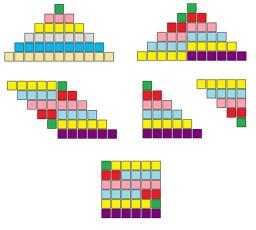Did you find an error or inaccuracy? Feel free to write us. Thank you!Tips to related online calculators
Do you have a linear equation or system of equations and looking for its solution? Or do you have a quadratic equation?

## Related math problems and questions:

• AS sequenceIn an arithmetic sequence is given the difference d = -3 and a71 = 455. a) Determine the value of a62 b) Determine the sum of 71 members.
• Difference AP 4Calculate the difference of the AP if a1 = 0.5, a2 + a3 = -1.1
• Fifth memberDetermine the fifth member of the arithmetic progression, if the sum of the second and fifth members equal to 73, and difference d = 7.
• Arithmetic progression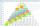In some AP applies: 5a2 + 7a5 = 90 s3 = 12 Find the first member a =? and difference d = ?
• Common difference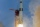The 4th term of an arithmetic progression is 6. If the sum of the 8th and 9th terms is -72, find the common difference.
• AP - simpleDetermine the first nine elements of sequence if a10 = -1 and d = 4
• Difference AP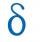Calculate the difference of arithmetic progression if the sum of its first 19 members Sn = 8075 and the first member is a1 = 20
• In aIn a triangle, the aspect ratio a: c is 3: 2, and a: b is 5: 4. The perimeter of the triangle is 74cm. Calculate the lengths of the individual sides.
• HP - harmonic progressionDetermine the 8th term of the harmonic progression 2, 4/3, 1,…
• Sequences AP + GPThe three numbers that make up the arithmetic sequence have the sum of 30. If we subtract from the first 5, from the second 4 and keep the third, we get the geometric sequence. Find AP and GP members.
• Third memberDetermine the third member of the AP if a4=93, d=7.5.
• ISO triangleCalculate the area of an isosceles triangle KLM if its sides' length is in the ratio k:l:m = 4:4:3 and has perimeter 377 mm.
• Chairs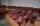Determine the number of seats in the seventh row and ninth row, if the 3rd row has 14 seats and in every next row of seats has five more than the previous row.
• GP - three membersThe second and third of a geometric progression are 24 and 12(c+1), respectively, given that the sum of the first three terms of progression is 76. determine the value of c.
• A rectangle 2A rectangle has a diagonal length of 74cm. Its side lengths are in ratio 5:3. Find its side lengths.
• Quotient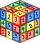Determine the quotient and the second member of the geometric progression where a3=10, a1+a2=-1,6 a1-a2=2,4.
• Negative difference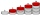Find four arithmetic progression members between 7 and -6.